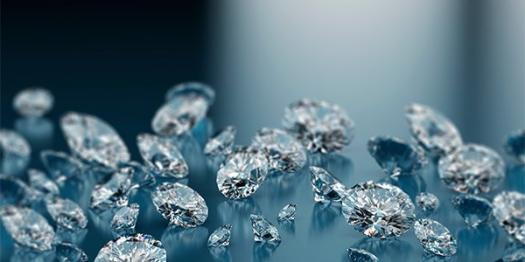Do You Know Covalent Crystal?

10 Questions | Total Attempts: 160SettingsIn covalent bond electron pairs are shared between atoms and in a Covalent crystal, atoms are connected with covalent bonds. How much do you know about this topic? Take this test and find out!

• 1.
Usually Covalent crystals have how many dimensions?
• A.

None

• B.

One dimension

• C.

Two dimensions

• D.

Three dimensions

• 2.
In what are Covalent crystalline solids soluble?
• A.

Polar solvents

• B.

Water

• C.

Non polar solvents

• D.

Normal saline

• 3.
What is like the structure of covalent compounds?
• A.

Closed

• B.

Open

• C.

Compact

• D.

Box like

• 4.
Which is one of non polar solvents?
• A.

Benzene

• B.

Potassium chromate

• C.

Normal saline

• D.

Water

• 5.
Which is the hardest Covalent Crystal?
• A.

Diamond

• B.

Graphite

• C.

Silicon carbide

• D.

Quartz

• 6.
How are Covalent crystals also called?
• A.

Rock solids

• B.

Network solids

• C.

Hard solids

• D.

Beautiful solids

• 7.
What is the stable phase of crystalline carbon under normal conditions (pressure, temperature)?
• A.

Quartz

• B.

Diamond

• C.

Graphite

• D.

Bucky Balls

• 8.
What is the melting point of silicon carbide?
• A.

1790 °C

• B.

2060 °C

• C.

2340 °C

• D.

2,730 °C

• 9.
What is the melting point of quartz?
• A.

1713 °C

• B.

1765 °C

• C.

1840 °C

• D.

1936 °C

• 10.
What is the melting point of diamond?
• A.

3220 °C

• B.

3550 °C

• C.

3660 °C

• D.

3770 °C

Related TopicsBack to top